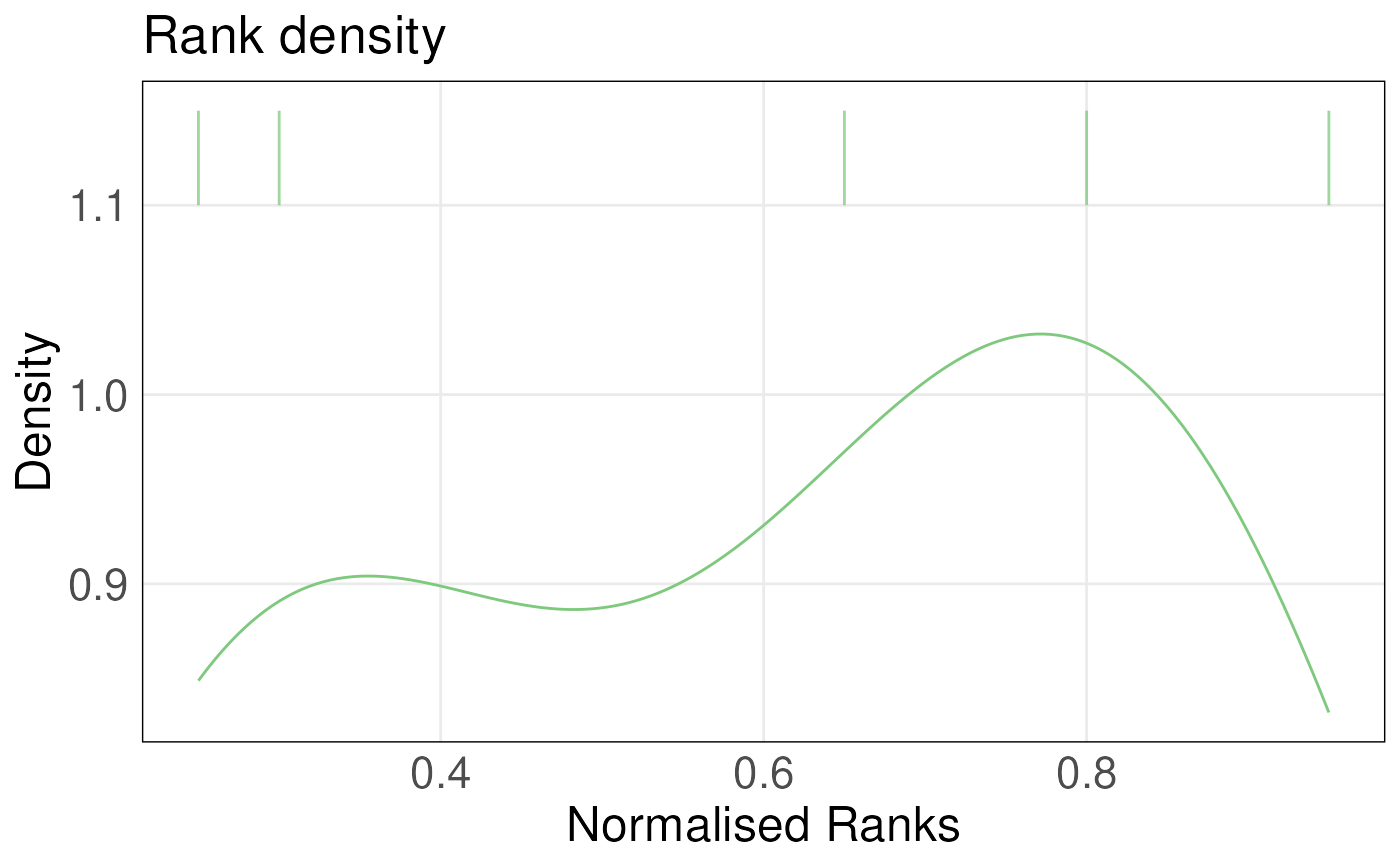This function takes a single-column data frame, which is a single-column subset of the ranked matrix data generated using rankGenes() function, and the gene sets of interest as inputs. It plots the density of ranks for genes in the gene set and overlays a barcode plot of these ranks. Ranks are normalized by dividing them by the maximum rank. Densities are estimated using KDE.

plotRankDensity(
rankData,
upSet,
downSet = NULL,
isInteractive = FALSE,
textSize = 1.5
)

# S4 method for ANY,vector,missing
plotRankDensity(
rankData,
upSet,
downSet = NULL,
isInteractive = FALSE,
textSize = 1.5
)

# S4 method for ANY,GeneSet,missing
plotRankDensity(
rankData,
upSet,
downSet = NULL,
isInteractive = FALSE,
textSize = 1.5
)

# S4 method for ANY,vector,vector
plotRankDensity(
rankData,
upSet,
downSet = NULL,
isInteractive = FALSE,
textSize = 1.5
)

# S4 method for ANY,GeneSet,GeneSet
plotRankDensity(
rankData,
upSet,
downSet = NULL,
isInteractive = FALSE,
textSize = 1.5
)

## Arguments

rankData one column of the ranked gene expression matrix obtained from the rankGenes() function, use drop = FALSE when subsetting the ranked gene expression matrix, see examples. GeneSet object or a vector of gene Ids, up-regulated gene set GeneSet object or a vector of gene Ids, down-regulated gene set Boolean, determine whether the returned plot is interactive numberic, set the size of text on the plot

## Value

A ggplot object (or a plotly object) with a rank density plot overlayed with a barcode plot

## Examples

ranked <- rankGenes(toy_expr_se)
plotRankDensity(ranked[,2,drop = FALSE], upSet = toy_gs_up)#> Warning: Ignoring unknown aesthetics: text